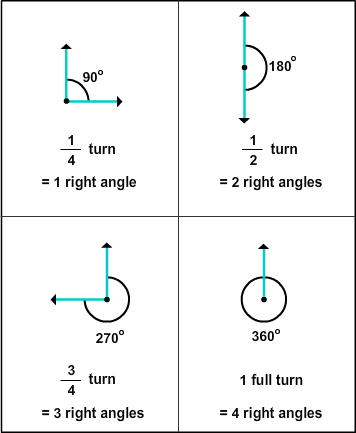# Angles of 90, 180, 270, and 360 Degrees

Rate 0 stars Common Core
Quiz size:
Message preview:
Someone you know has shared Angles of 90, 180, 270, and 360 Degrees quiz with you:

To play this quiz, click on the link below:

https://www.turtlediary.com/quiz/angles-of-90-180-270-and-360-degrees.html

Hope you have a good experience with this site and recommend to your friends too.

Login to rate activities and track progress.
Login to rate activities and track progress.

We can find the measure of a given angle by relating turns to right angles.For example, consider the angle given below.It is
3 4
turn.

So, the measure of this angle is equal to 3 right angles or 270°.

ds

A B C D E F G H I J K L M N O P Q R S T U V W X Y Z

### Help

##### Remember :

The smallest number is the one that comes first while counting.

##### Solution :

To arrange the given numbers in order from smallest to greatest, find the smallest number among all the given numbers.

21,27,23

21 is the smallest number.